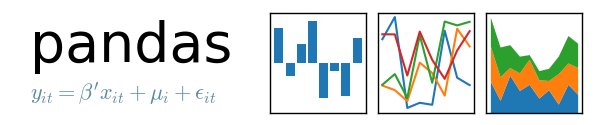# Vectorized Operations and Sorting in Pandas Series

Last updated: July 8th, 2019# Vectorized operations and sorting in Pandas Series¶

Series also support vectorized operations and aggregation functions as Numpy, on this lecture we'll see most common ones.## Hands on!¶

In [ ]:
import pandas as pd
import numpy as np

In [ ]:
pd.options.display.float_format = '{:,.2f}'.formatThe first thing we'll do is create again the Series from our previous lecture:

In [ ]:
g7_pop = pd.Series({
'France': 63.951,
'Germany': 80.94,
'Italy': 60.665,
'Japan': 127.061,
'United Kingdom': 64.511,
'United States': 318.523
}, dtype=np.float, name='G7 Population in millions')

In [ ]:
g7_pop

In [ ]:
gdp = pd.Series(
[1785387, 2833687, 3874437, 2167744, 4602367, 2950039, 17348075],
'Japan', 'United Kingdom', 'United States'],
dtype=np.float,
name='G7 GDP in millions')

In [ ]:
gdp

In [ ]:
g7_pop.head(3)

In [ ]:
g7_pop.tail(3)## Series vectorized operations¶

In [ ]:
g7_pop * 1_000_000

In [ ]:
g7_pop + 1_000_000

In [ ]:
gdp * 1_000_000


Operation between Series:

In [ ]:
gdp / g7_pop

In [ ]:
(gdp * 1_000_000) / (g7_pop * 1_000_000)## Using Universal Functions (Ufuncs) to obtain statistical info¶

We can apply any Universal Function to a Series.

Another useful method is describe, which gives you a good "summary" of the Series. Let's explore other methods in more detail:

In [ ]:
g7_pop.describe()

In [ ]:
g7_pop.max()

In [ ]:
g7_pop.min()

In [ ]:
g7_pop.mean()

In [ ]:
g7_pop.std()

In [ ]:
g7_pop.quantile(.2)

In [ ]:
g7_pop.quantile(.8)

In [ ]:
np.log(g7_pop)## Sorting Series values¶

In many use cases Series values need to be sorted.

Sorting in Pandas is extremely easy. There are two important methods to be used for Series and DataFrames that will take care of the job: sort_values and sort_index.

In [ ]:
g7_pop

In [ ]:
g7_pop.sort_values()


As you can see, sorting is as simple as invoking the sort_values method. By default, values are sorted in ascending order, which you can customize with the ascending parameter.

In [ ]:
g7_pop.sort_values(ascending=False)

In [ ]:
g7_pop

In [ ]:
g7_pop.sort_values(ascending=False, inplace=True)

In [ ]:
g7_pop


### Sorting index¶

sort_index works exactly in the same way:

In [ ]:
g7_pop.sort_index()